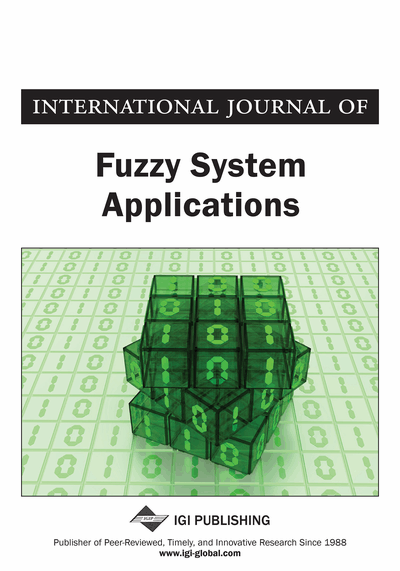# Bounded and Semi Bounded Inverse Theorems in Fuzzy Normed Spaces

DOI: 10.4018/IJFSA.2015040104
Available
\$29.50
No Current Special Offers

## Abstract

In this paper, the author introduces the notion of the complete fuzzy norm on a linear space. And the author considers some relations between the fuzzy completeness and ordinary completeness on a linear space, moreover a new form of fuzzy compact spaces, namely b-compact spaces, b-closed space is introduced. Some characterization of their properties is obtained. Also some basic properties for linear operators between fuzzy normed spaces are further studied. The notions of fuzzy vector spaces and fuzzy topological vector spaces were introduced in Katsaras and Liu (1977). These ideas were modified by Katsaras (1981), and in (1984) Katsaras defined the fuzzy norm on a vector space. In (1991) Krishna and Sarma discussed the generation of a fuzzy vector topology from an ordinary vector topology on vector spaces. Also Krishna and Sarma (1992) observed the convergence of sequence of fuzzy points. Rhie et al. (1997) Introduced the notion of fuzzy a-Cauchy sequence of fuzzy points and fuzzy completeness.
Article Preview
Top

## 1. Introduction And Preliminaries

The notions of fuzzy vector spaces and fuzzy topological vector spaces were introduced in Katsaras and Liu (1977). These ideas were modified by Katsaras (1981), and in (1984) Katsaras defined the fuzzy norm on a vector space. In (1991) Krishna and Sarma discussed the generation of a fuzzy vector topology from an ordinary vector topology on vector spaces. Also Krishna and Sarma (1992) observed the convergence of sequence of fuzzy points. Rhie et al. (1997) Introduced the notion of fuzzy Cauchy sequence of fuzzy points and fuzzy completeness.

In this paper, we first observe a type of the convergence of sequences as an analogy of Bag and Samanta (2003) in a fuzzy normed linear space. Secondly, we introduce the notion of a complete fuzzy norm, using the convergence of a sequence of a linear space. And we consider some relations between the fuzzy completeness and the ordinary completeness on a linear space.

## Complete Article List

Search this Journal:
Reset
Volume 12: 1 Issue (2023)
Volume 11: 4 Issues (2022)
Volume 10: 4 Issues (2021)
Volume 9: 4 Issues (2020)
Volume 8: 4 Issues (2019)
Volume 7: 4 Issues (2018)
Volume 6: 4 Issues (2017)
Volume 5: 4 Issues (2016)
Volume 4: 4 Issues (2015)
Volume 3: 4 Issues (2013)
Volume 2: 4 Issues (2012)
Volume 1: 4 Issues (2011)
View Complete Journal Contents Listing